### Home > CALC > Chapter 4 > Lesson 4.1.1 > Problem4-7

4-7.
1.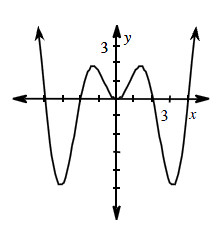Examine f(x) graphed at right. Homework Help ✎

1. Is f(x) even, odd, or neither?

2. If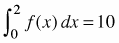, what is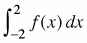? Explain.

3. If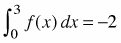and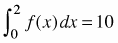find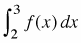. Explain.

4. If you know that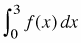and, how can you find? Justify your process with a diagram, if necessary.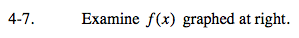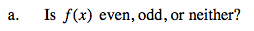Even functions have reflective symmetry across the y-axis. Odd functions have rotational symmetry about the origin.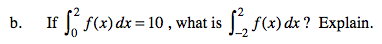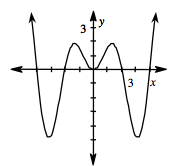$\int_{0}^{2}f(x)dx = 10 \text{ means the exact area under }f(x)\text{ between }x=-2 \text{ and }x=2\text{ is }10.$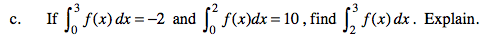$\int_{2}^{3}f(x)dx=\int_{0}^{3}f(x)dx-\int_{0}^{2}f(x)dx=$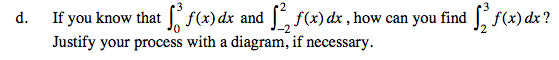Refer to the steps in part (c) for more guidance.